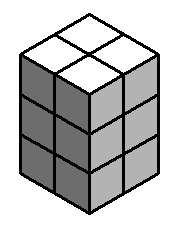# MathsClass

A blog about teaching and learning in a maths classroom.

## Teaching Surface Area

Thursday, 25 November 2010 | 1 Comment

I like teaching surface area, I think it’s an interesting topic. Yet, I find kids struggle with the concept. Not understanding the basics of area and then getting over the prior knowledge of solids meaning volume are two aspects that cause some difficulty.

This is how I break down surface area for my low ability Year 8 class.### Lesson 1 – Introducing Surface Area

1. Investigate Surface Area using Centicubes (comparing with Volume).
Concepts: counting the faces of the centicubes rather than counting the cubes, reinforced by creating multiple solids with different surface areas using the same number of cubes, understand what is meant by a 3 × 2 × 2 rectangular prism. Layed out in “Making Solids: Investigation” (Page 1 of download below). This is based on an activity in the book Access to Stage 5.1 Maths.
1. From a net, make a solid and find the surface area.
Concepts: calculating area by counting squares, see that a rectangular prism is made up of six rectangles (3 pairs of congruent rectangles). Make net (Page 3 of download below). Print to card, and cut in half (no tabs on these nets, I use sticky tape to hold together).
1. Find the surface area by drawing a net and counting squares.
Similar to 2., but this time students draw their own net. Concepts: the drawing of a net from an image of a solid, understand how the edges of the rectangles join. Draw nets – Page 4 of download below.
1. Find the surface area from nets where the area of each face is shown.

### Lesson 2 – Rectangular Prisms

1. Find the surface area of solids where the area of some faces shown (e.g. show the front, top and side of a rectangular prism).

2. Concepts: understand that the faces of a rectangular prism come in pairs.
3. Find the surface area of a cube given the length of a single edge.
1. Find the surface area of a rectangular prism given the length of 3 edges.

Concepts: determine which lengths are used for each face and that faces have a matching face so the area of a face can be doubled.

### Lesson 3 – Triangular Prisms

1. Review area.
1. Students draw a 3cm square and find it’s area. Then, draw a diagonal and find the area of the resulting triangle(s).
2. Students draw a 4 by 5cm rectangle and find it’s area. Then, draw a diagonal and find the area of the resulting triangle(s).
1. Determine a means for finding the area of a triangle.
1. Make two triangular prisms.
Concepts: understand how a triangular prism is constructed from two triangles and 3 rectangles, understand how the side lengths of the triangles relate to the side lengths of the rectangles, see the common side length of all the rectangles. Use: Paper Model of a Triangular Prism
1. Show my triangular prism t-shirt box for which I’ve made peel off sides, peel off each side and form the net.
1. Complete some exercises given diagrams of triangular prisms with side lengths.

### Lesson 4 – Smarties

1. Determine the surface area of a Smarties box
1. Design a Toblerone box

Posted in • Lesson IdeaSurface AreaPrintableWorksheet | Short URL: http://mths.co/2047

• Share:

Nordin Zuber on  29 November 10  at  10:43 AM #
Got to love any activities that uses Smarties or Toblerone 😊 I'm doing one tomorrow to build a frequency histogram of the colour of the Smarties in the box.

Commenting is not available in this channel entry.

New  Subscribe to the …

Simon Job — eleventh year of teaching maths in a public high school in Western Sydney, Australia.
MathsClass is about teaching and learning in a maths classroom. more→

## Elsewhere@simonjob
updates via@mathslinks